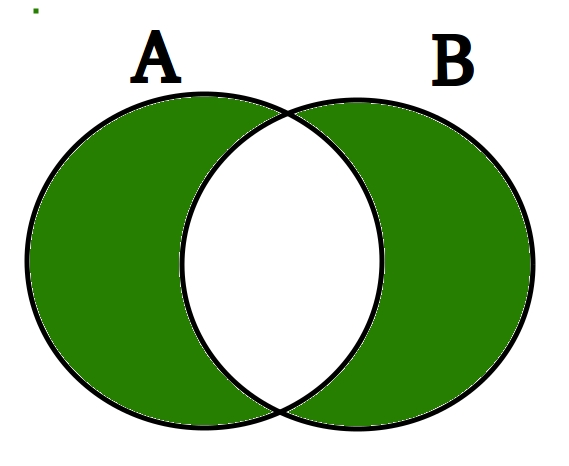Related Articles

# Python Set symmetric_difference()

• Difficulty Level : Easy
• Last Updated : 06 Feb, 2018

The symmetric difference of two sets set1 and set2 is the set of elements which are in either of the sets set1 or set2 but not in both.Syntax :

`set1_name.symmetric_difference(set2_name) `

Parameters :
It only takes a single set as the parameter. If a list, tuple or dictionary is passed it converts it a set and performs the task.

Return value :

`Returns a set which is the symmetric difference between the two sets. `

Working Code for symmetric_difference() :

 `# Python program to demonstrate the use of ``# of symmetric_difference() method `` ` ` ` `list1 ``=` `[``1``, ``2``, ``3``] ``list2 ``=` `[``2``, ``3``, ``4``] ``list3 ``=` `[``3``, ``4``, ``5``] `` ` `# Convert list to sets``set1 ``=` `set``(list1) ``set2 ``=` `set``(list2) `` ` `# Prints the symmetric difference when  ``# set is passed as a parameter ``print``(set1.symmetric_difference(set2)) `` ` `# Prints the symmetric difference when list is ``# passed as a parameter by converting it to a set``print``(set2.symmetric_difference(list3))`

Output :

```{1, 4}
{2, 5}
```

Attention geek! Strengthen your foundations with the Python Programming Foundation Course and learn the basics.

To begin with, your interview preparations Enhance your Data Structures concepts with the Python DS Course. And to begin with your Machine Learning Journey, join the Machine Learning – Basic Level Course

My Personal Notes arrow_drop_up